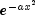JMSLTM Numerical Library 7.2.0
com.imsl.math

• All Implemented Interfaces:
Enclosing class:

```public static class RadialBasis.Gaussian
extends Object
The Gaussian basis function,.
Example
• ### Constructor Summary

Constructors
Constructor and Description
`RadialBasis.Gaussian(double a)`
Creates a Gaussian basis function.
• ### Method Summary

Methods
Modifier and Type Method and Description
`double` `f(double x)`
A Gaussian basis function.
`double` `g(double x)`
The derivative of the Gaussian basis function used to calculate the `gradient` of the radial basis approximation.
• ### Methods inherited from class java.lang.Object

`clone, equals, finalize, getClass, hashCode, notify, notifyAll, toString, wait, wait, wait`
• ### Constructor Detail

`public RadialBasis.Gaussian(double a)`
Creates a Gaussian basis function.
Parameters:
`a` - a `double` specifying the value of the function parameter. Decreasing the Gaussian parameter decreases fitting-error but may increase computational effort.
• ### Method Detail

• #### f

`public double f(double x)`
A Gaussian basis function.
Specified by:
`f` in interface `RadialBasis.Function`
Parameters:
`x` - a `double`, the point at which the function is to be evaluated
Returns:
a `double`, the value of the function at x
• #### g

`public double g(double x)`
The derivative of the Gaussian basis function used to calculate the `gradient` of the radial basis approximation.
Specified by:
`g` in interface `RadialBasis.Function`
Parameters:
`x` - a `double`, the point at which the function is to be evaluated
Returns:
a `double`, the value of the function at x
JMSLTM Numerical Library 7.2.0